## Fibonacci n-Step Number

An-step Fibonacci sequence is given by definingfor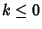,,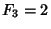, and(1)

for. The casecorresponds to the degenerate 1, 1, 2, 2, 2, 2 ...,to the usual Fibonacci Numbers 1, 1, 2, 3, 5, 8, ... (Sloane's A000045),to the Tribonacci Numbers 1, 1, 2, 4, 7, 13, 24, 44, 81, ... (Sloane's A000073),to the Tetranacci Numbers 1, 1, 2, 4, 8, 15, 29, 56, 108, ... (Sloane's A000078), etc.

The limitis given by solving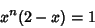(2)

forand taking the Real Root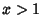. If, the equation reduces to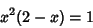(3)(4)

giving solutions(5)

The ratio is therefore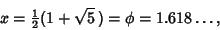(6)

which is the Golden Ratio, as expected. Solutions for, 2, ... are given numerically by 1, 1.61803, 1.83929, 1.92756, 1.96595, ..., approaching 2 as.

Sloane, N. J. A. Sequences A000045/M0692, A000073/M1074, and A000078/M1108 in An On-Line Version of the Encyclopedia of Integer Sequences.'' http://www.research.att.com/~njas/sequences/eisonline.html and Sloane, N. J. A. and Plouffe, S. The Encyclopedia of Integer Sequences. San Diego: Academic Press, 1995.